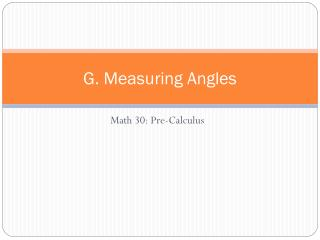DownloadDownload PresentationG. Measuring Angles

G. Measuring Angles

Télécharger la présentationG. Measuring Angles

- - - - - - - - - - - - - - - - - - - - - - - - - - - E N D - - - - - - - - - - - - - - - - - - - - - - - - - - -
Presentation Transcript

1. G. Measuring Angles Math 30: Pre-Calculus

2. PC30.1 • Extend understanding of angles to angles in standard position, expressed in degrees and radians. • PC30.2 • Demonstrate understanding of the unit circle and its relationship to the six trigonometric ratios for any angle in standard position. • PC30.4 • Demonstrate understanding of first- and second-degree trigonometric equations.

3. Key Terms:

4. 1. Angles and Measuring Angles • PC30.1 • Extend understanding of angles to angles in standard position, expressed in degrees and radians.

5. 1. Angles and Measuring Angles

6. So to convert between the two measurement systems we can use the following to covertions. • Degrees to radians • Radians to degrees

7. It is acceptable to omit the radians at the end. So instead of 2π/3 radians we can just write 2π/3 which is understood to be radians.

8. Example 1

9. When you sketch a 60º and 420º angle in standard position, the terminal arms coincide. These are Coterminal Angles.

10. Example 2

11. For example what are angles coterminal with: • 40º • 2π/3

12. Example 3

13. All arcs subtending a right angle (π/2) have the same central angle, but different arc lengths depending on the radius. Arc length is proportional to the radius. • This is true for any central circle

14. Consider the 2 concentric circles. • Radius of small circle is 1 and the larger circle is r. • A central angle of θrads in a subtended arc. AB on smaller circle and CD on larger circle. • We can write a proportion, when x represents the arc length of the small circle and a arc length of the larger circle.

15. Consider the circle with r=1 and central angle θ • The ratio of the arc length to the circumference is equal to the ratio of the central angle to one full rotation.

16. a=θr • This formula, works for any circle, provided θ is in rads and both a and r are in the same units.

17. Radians are especially useful for describing circular motion. • Arc length, a, means the distance travelled along the circumference of a circle of radius r. • For a central angle θ, in radians, a=θr

18. Example 4

19. Key Ideas p.175

20. Practice • Ex. 4.1 (p.175) #1-8 odds in each, 9,10, 11-13 odds in each, 14-22 evens #3,5,6,7,9,11,12,13 odds in each, 15-27 odds

21. 2. Unit Circle • PC30.2 • Demonstrate understanding of the unit circle and its relationship to the six trigonometric ratios for any angle in standard position.

22. 2. Unit Circle

23. A unit circle is drawn on a Cartesian plane with the center at the origin and has a radius of 1 unit (not necessarily a Cartesian plane but that is how we will be using it)

24. We can find the equation of the unit circle using teh Pythagorean Theorem.

25. Example 1

26. Example 2

27. The formula a=θr applies to any circle as long as a and r are in the same units • In the unit circle r=1 so the formula simplifies to a=θ(1) or a=θ • This means the central angle and its subtended arc on the circle have the same value.

28. You can use the function P(θ)=(x,y) to link arc length, θ, of a central angle in a unit circle to the coordinates, (x,y) of the point of intersection of the terminal arm and unit circle. • If we join P(θ) to the origin we create a central angle θ in standard position • The central angle and arc length are both θ radians and θ units respectively.

29. Function P, takes real number values for central angles or arc length on the unit circle and matches them with specific points. • For example, if θ=π, then point (-1,0). Thus, we write P(π)=(-1,0)

30. Example 3

31. Key Ideas p. 186

32. Practice • Ex. 4.2 (p.186) #1-5 odds in each, 6, 7, 9-15 odds #3-5 odds in each,6,7,9, 10-20 evens

33. 3. Trig Ratios • PC30.2 • Demonstrate understanding of the unit circle and its relationship to the six trigonometric ratios for any angle in standard position.

34. 3. Trig Ratios • For this section we will have to recall come prior knowledge. • What are the three trig ratios that you know?

35. Recall a unit circle has a radius of 1 unit. • Also with a unit circle P(θ)=(x,y) where P is a point on the circumference of the circle.

36. There are 3 more Trig ratios that we have not looked at before and they are called the Reciprocal Trig Ratios • They are the reciprocals of sine, cosine and tangent. • They are called cosecant, secant, and cotangent.

37. Example 1

38. Exact values for the Trig ratios can be determined using the special triangles and multiples of θ=0, π/6, π/4, π/3, π/2or θ=0º, 30º, 45º, 60º, 90ºfor points P(θ) on the unit circle.

39. Example 2